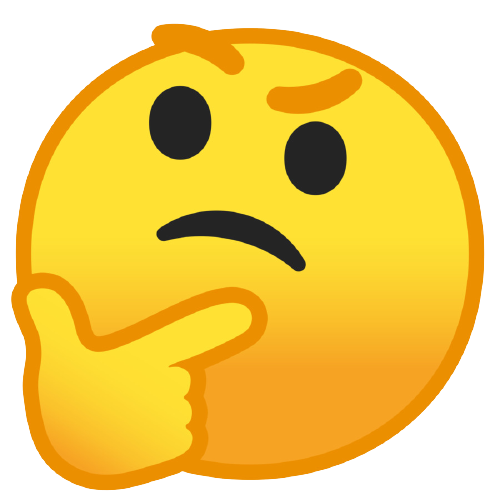Related Tags

python
quantum computing
course related

# What are cloning transformations?Abdul Mateen

Quantum computing is radically altered from classical computing. To become a pro in quantum computing, you must ignore discoveries of classical computing.

In classical computing, it is evident that we are dealing with the stateless bitclassical bit, which is either 0 or 1. However, in quantum computing, a quantum bitqubit is not 0 or 1. The qubit is in a complex linear combination of 0 and 1’s that also includes states.

The classical computer provides us the privilege to interact with the classical bit without damaging it. Although in quantum computing, when we interact with the quantum bit, we will hurt it somehow. More precisely, it is impossible to capture the information perfectly from a quantum bit if its initial state is unknown.

But that doesn’t mean it is not measurable or that we cannot get the qubit’s state. Instead, the issue is that when we try to measure it, the quantum superposition collapses.

This is frequently misunderstood to imply that any interaction with a quantum entity annihilates it. But, a better way to think about it is that the exchange could put miles on the quantum entity. There will be some wear and tear, and that may destroy it.Suppose two qubits can yield a state of superposition. Once you measure one qubit, its entangled fellow immediately jumps to a different state of superposition. Even if it is light-years away, it seems to know a measurement has taken place, and it takes on a state that acknowledges the measured value.

Wouldn’t it be great to create a copy of the qubit before we measure it? Then, we would measure one copy of the qubit while continuing to work with the other copy.

The illustration below draws the picture of a cloning transformation gate.

The Cloning Gate

The gate G takes an arbitrary qubit |ψ⟩ and a fixed |0⟩ (an ancilla bit) input. It outputs two copies of |ψ⟩. Below are three examples of cloning transformations:

1. It clones a qubit in the state |0⟩
G(|0⟩|0⟩)=|0⟩|0⟩
1. It clones a qubit in the state |1⟩
G(|1⟩|0⟩)=|1⟩|1⟩
1. It clones an arbitrary qubit |ψ⟩
G(|ψ⟩|0⟩)=|ψ⟩|ψ⟩According to the *No Cloning Theorem, it is impossible to create an independent and identical copy of an arbitrary qubit.

So far, we have figured out that perfect cloning is not possible. However, it is possible to perform imperfect cloningwhere the copies have a non-perfect fidelity.

For that, we need to find one unitary that would work for all states because the no-cloning theorem only addresses the copying of an unknown quantum state. We can this unitary from scratch without using the prototype qubit if we know exactly what state we need to create.

Here comes the new gate that is Transformation O Gate.

The following illustration illustrates the transformation gate $O_{i}$:

The Transformation Gate

This $O_{i}$ gate transforms an arbitrary quantum state |x⟩ into the quantum state |$f_{i}$(x)⟩ - the function $f_{i}$ given the input x.

Since $O_{i}$​​​ is a quantum transformation gate, it must be reversible. Therefore, it must have the same size of input and output, and each result must be uniquely identified from the information it originates. This means the Transformation O Gate can provide us unitary that would work for all states, and then we can clone the qubit imperfectly.

### Cloning transformation?

Based on all the information above, we can say that the cloning transformation is a process of transformation that can use to clone the quantum entityQubit imperfectly.

If the transformation is chosen correctly, the qubit will approximate its clone of the original qubit.

### Pragmatic Example

Cloning Transformation can be used as an eavesdropping attack on quantum cryptography protocols.

It is impossible to copy data encoded in a quantum state. If one attempts to read the encoded data, it will change the quantum state (no-cloning theorem).

Take a look at the illustration below.

### Example

Below is the practical example of Cloning Transformation in Python.

# importing the copy, projectq package.
from projectq import MainEngine
from projectq.ops import All, CNOT, H, Measure, X, Z

# initilizing the qubits.
quantum_engine = MainEngine()
qubit1 = quantum_engine.allocate_qubit()
qubit2 = quantum_engine.allocate_qubit()

# applying the Hadamard and CNOT (Transformation) gates to the Qubit.
H | qubit1
CNOT | (qubit1, qubit2)

# initilizing the qubit for cloning qubit 1 to 2.
qubit_to_clone = quantum_engine.allocate_qubit()

# applying the Pauli-X, CNOT, Hadamrd (Transformation) gates to the Qubit to clone.
X | qubit_to_clone
CNOT | (qubit_to_clone, qubit1)
H | qubit_to_clone

# measuring the qubits
Measure | qubit_to_clone
Measure | qubit1

# crating list for checking
cloning = [int(qubit_to_clone), int(qubit1)]

# checking the states of qubits (original and clonned qubit).
if cloning == 1:
# fliping the base state by applying a Pauli-X gate.
X | qubit2
if cloning == 1:
# applying a Pauli-Z gate that leaves state |0⟩ unchanged, but flips |1⟩ to |-1⟩.
Z | qubit2
# measuring the qubits if all went well qubit2 have same value as of qubit1
Measure | qubit2

print("The value of Original Qubit: ",int(qubit1))
print("The value of Qubit used for Cloning Purpose: ",int(qubit_to_clone))
print("The value of Qubit that is Clone of original Qubit: ",int(qubit2))

This is a simple example for cloning transformation in Python using simple quantum gates.

RELATED TAGS

python
quantum computing
course related

CONTRIBUTORAbdul Mateen